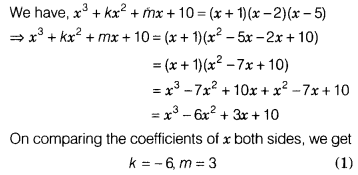# If x3 + kx2 +mx +10 =(x + i)(x -2)(x-5), then find the values of k and m

If x3 + kx2 +mx +10 =(x + 1)(x -2)(x-5), then find the values of k and m.